# Common Core: High School - Geometry : Arc Length, Radii, Radian, and Sector Similarity and Proportionality: CCSS.Math.Content.HSG-C.B.5

## Example Questions

← Previous 1 3

### Example Question #1 : Arc Length, Radii, Radian, And Sector Similarity And Proportionality: Ccss.Math.Content.Hsg C.B.5

If the radius is, and the central angle is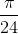find the arc length.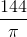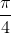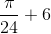Explanation:

To find the arc length, we simply multiply the radius by the central angle.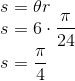### Example Question #1 : Arc Length, Radii, Radian, And Sector Similarity And Proportionality: Ccss.Math.Content.Hsg C.B.5

If the radius is, and the central angle is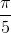find the arc length.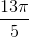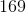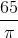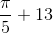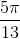Explanation:

To find the arc length, we simply multiply the radius by the central angle.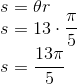### Example Question #51 : Circles

If the radius is, and the central angle is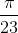find the arc length.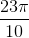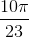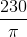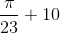Explanation:

To find the arc length, we simply multiply the radius by the central angle.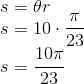### Example Question #1 : Arc Length, Radii, Radian, And Sector Similarity And Proportionality: Ccss.Math.Content.Hsg C.B.5

If the radius is, and the central angle isfind the arc length.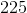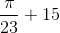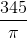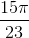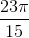Explanation:

To find the arc length, we simply multiply the radius by the central angle.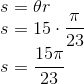### Example Question #2 : Arc Length, Radii, Radian, And Sector Similarity And Proportionality: Ccss.Math.Content.Hsg C.B.5

If the radius is, and the central angle is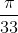find the arc length.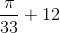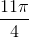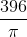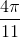Explanation:

To find the arc length, we simply multiply the radius by the central angle.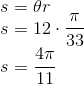### Example Question #1 : Arc Length, Radii, Radian, And Sector Similarity And Proportionality: Ccss.Math.Content.Hsg C.B.5

If the radius is, and the central angle is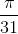find the arc length.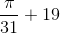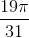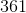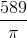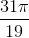Explanation:

To find the arc length, we simply multiply the radius by the central angle.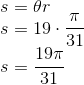### Example Question #2 : Arc Length, Radii, Radian, And Sector Similarity And Proportionality: Ccss.Math.Content.Hsg C.B.5

If the radius is, and the central angle is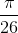find the arc length.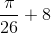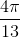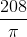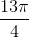Explanation:

To find the arc length, we simply multiply the radius by the central angle.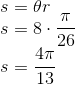### Example Question #8 : Arc Length, Radii, Radian, And Sector Similarity And Proportionality: Ccss.Math.Content.Hsg C.B.5

If the radius is, and the central angle isfind the arc length.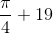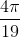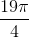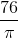Explanation:

To find the arc length, we simply multiply the radius by the central angle.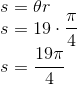### Example Question #9 : Arc Length, Radii, Radian, And Sector Similarity And Proportionality: Ccss.Math.Content.Hsg C.B.5

If the radius is, and the central angle is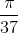find the arc length.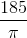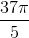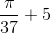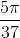Explanation:

To find the arc length, we simply multiply the radius by the central angle.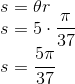### Example Question #10 : Arc Length, Radii, Radian, And Sector Similarity And Proportionality: Ccss.Math.Content.Hsg C.B.5

If the radius is, and the central angle isfind the arc length.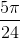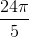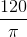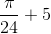Explanation:

To find the arc length, we simply multiply the radius by the central angle.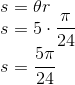← Previous 1 3

### All Common Core: High School - Geometry Resources